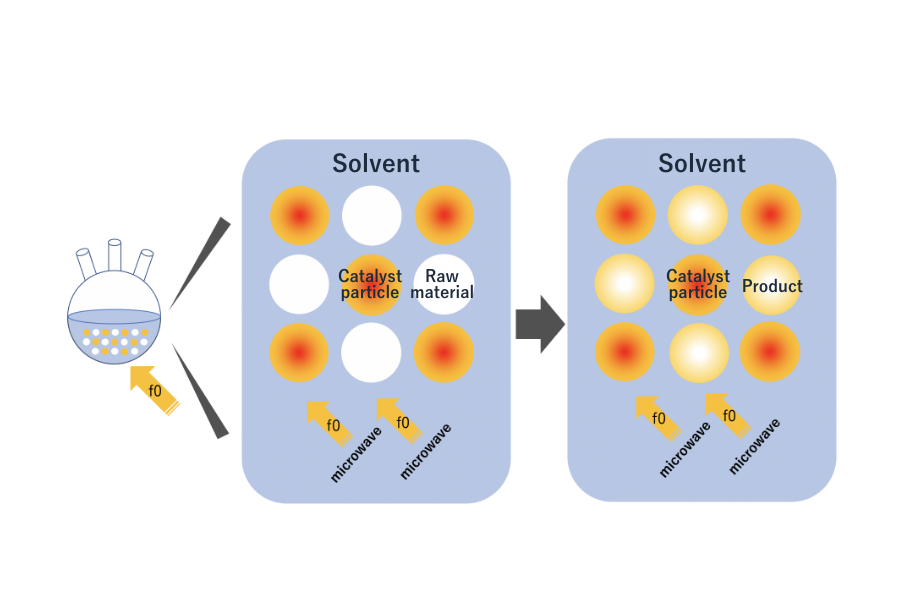Flow – 01

# Reaction System Design I– Reaction Conditions Suitable for Microwaves

## Microwave Absorption Capacity VerificationFigure 1: Complex dielectric constant measurement device

The most important element for microwave chemistry is knowing how much each molecule absorbs the microwaves. The absorption rate of microwaves by a molecule is expressed in terms of the complex dielectric constant ε”. For this reason, all of our research and development begins by measuring the complex dielectric constant ε” using the equipment shown in Figure 1.Figure 2: Relationship between complex dielectric constant ε’’ and the heating capacity of a molecule heated by microwaves.

As shown in Figure 2, microwaves fully penetrate materials with small ε” values but are absorbed by materials with large ε’’ values. As a result, selective heating only works for molecules with high ε’’ values.
This heating phenomenon is called dielectric heating as it occurs as a result of the interaction between microwaves and the molecules that comprise the material.Figure 3: Microwave heating rate per unit volume of material

In addition to dielectric heating, conductive heating and magnetic heating are other methods for heating materials with microwaves. Dielectric heating occurs when microwaves interact with polarized matter such as water or alcohol while conductive heating happens through the interaction with metals and magnetic heating with materials as ferrites. Generally speaking, the microwave heating rate Ptotal (W/m3) per unit volume of material may be expressed as shown in Figure 3, where Pε, Pσ, and Pμ represent the dielectric, conductive, and magnetic heating rates, respectively.

## Frequency and Temperature Dependence of Complex Dielectric ConstantFigure 4: The complex dielectric constant of ethylene glycol as a function of frequency and temperature. The vertical axis is the complex dielectric constant ε” and the horizontal axis is the frequency (GHz). Each colored line represents a different temperature (20-180 °C).

The complex dielectric constant varies with the temperature of the material and the frequency of the irradiated microwaves.

Figure 4 plots the complex dielectric constant of ethylene glycol at frequencies ranging between 0.1-20 GHz and temperatures of 20-180 °C. For example, focusing on the 2.45 GHz band used in commercial microwave ovens (the dotted line), we can see that the complex dielectric constant of ethylene glycol decreases as the temperature rises from 20 °C to 180 °C. The higher the temperature, the lower the complex dielectric constant and the further the peak of the complex dielectric constant shifts to the high frequency side.

Because the dependence on frequency and temperature differs with each molecule, understanding the complex dielectric constant of each molecule as a function of temperature and frequency is crucial when designing a reaction system.

## Complex Dielectric Constant Library

We have compiled a library of data on complex dielectric constants measured as a function of temperature and frequency (Figure 5) and use this data to select temperatures and microwave frequencies that allow heated materials to absorb microwave energy most efficiently.

Figure 5: Complex dielectric constant library

## Designing a Reaction System

The following is a brief introduction on how we proceed with designing a reaction system.

As Figure 6 shows, we consider the reactions necessary to obtain a product by irradiating microwaves to a system containing raw materials, catalyst particles, and solvents. For example, let us assume that the complex dielectric constant measurements of each material are those shown in Figure 7. In this case, the raw material absorbs almost no microwaves, but the catalyst particles and the solvent show high absorption levels at frequencies f0 and f2. Heating the solvent will heat the entire system, just as the same with conventional methods. So, in this system, the catalyst particles are selectively heated by microwave irradiation at frequency f0, and they will heat the raw materials as their temperature rises. In other words, the raw materials are heated via the catalyst particles.

In this example, the catalyst particles are selectively heated, creating a state in which the temperature of the catalyst particles is higher than that of the source material. Conventional heating methods cannot create such conditions because they heat the entire system. Microwave heating creates a temperature difference between the catalyst particles and the source material, thereby shortening chemical reaction time and increasing product yield compared to conventional heating methods. Differences in temperature can be adjusted by changing the amount of microwave irradiation energy. This high degree of flexibility in operating conditions is yet another feature of the microwave heating method.

• Figure 6: Reaction diagram of a system consisting of raw materials, catalyst particles, and solvent irradiated by microwaves to obtain a product.
• Figure 7: Schematic diagram of the complex dielectric constant of catalyst particles, raw material, and solvent in the system shown in Figure 6.